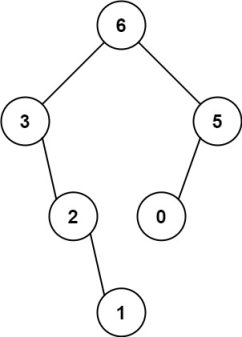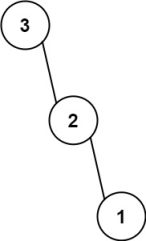### 题意输入：nums = [3,2,1,6,0,5]

- [3,2,1,6,0,5] 中的最大值是 6 ，左边部分是 [3,2,1] ，右边部分是 [0,5] 。
- [3,2,1] 中的最大值是 3 ，左边部分是 [] ，右边部分是 [2,1] 。
- 空数组，无子节点。
- [2,1] 中的最大值是 2 ，左边部分是 [] ，右边部分是  。
- 空数组，无子节点。
- 只有一个元素，所以子节点是一个值为 1 的节点。
- [0,5] 中的最大值是 5 ，左边部分是  ，右边部分是 [] 。
- 只有一个元素，所以子节点是一个值为 0 的节点。
- 空数组，无子节点。输入：nums = [3,2,1]

• 1 <= nums.length <= 1000
• 0 <= nums[i] <= 1000
• nums 中的所有整数 互不相同

### 题解：dfs

• 如果l>r：则构造不了，返回空二叉树
• 否则的话，寻找该区间中最大值，然后再递归的构造其左子树和右子树

#### C++代码

/**
* Definition for a binary tree node.
* struct TreeNode {
*     int val;
*     TreeNode *left;
*     TreeNode *right;
*     TreeNode() : val(0), left(nullptr), right(nullptr) {}
*     TreeNode(int x) : val(x), left(nullptr), right(nullptr) {}
*     TreeNode(int x, TreeNode *left, TreeNode *right) : val(x), left(left), right(right) {}
* };
*/
class Solution {
public:
TreeNode* constructMaximumBinaryTree(vector<int>& nums) {
return dfs(nums,0,nums.size()-1);
}
TreeNode* dfs(vector<int>& nums,int l,int r){
if(l>r) return nullptr;
int pos=l,maxnum=nums[l];
for(int i=l+1;i<=r;i++){
if(nums[i]>maxnum) maxnum=nums[i],pos=i;
}
TreeNode* root=new TreeNode();
root->val=maxnum;
root->left=dfs(nums,l,pos-1);
root->right=dfs(nums,pos+1,r);
return root;
}
};